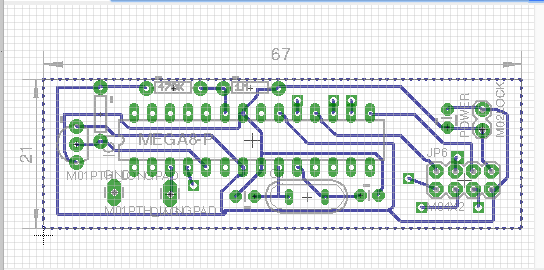# Battery percentage incorrectly send to gateway.

• I ordered a custom pcb with a ATMega328P and a dallas tempsensor , powered by 2AA batteries. Simular like http://forum.mysensors.org/topic/1107/sensors-in-boxes/7.

I use allso the sketch as shown in that topic, however the battery status allways read 102%. I'm sure the batteries are not full.
Can you point me in the right direction why my battery percentage is not send correctly?

// Example sketch showing how to send in OneWire temperature readings
#include <MySensor.h>
#include <SPI.h>
#include <DallasTemperature.h>
#include <OneWire.h>

#define ONE_WIRE_BUS 3 // Pin where dallase sensor is connected
#define MAX_ATTACHED_DS18B20 16
unsigned long SLEEP_TIME = 30000; // Sleep time between reads (in milliseconds)
OneWire oneWire(ONE_WIRE_BUS);
DallasTemperature sensors(&oneWire);
MySensor gw;
float lastTemperature[MAX_ATTACHED_DS18B20];
int numSensors=0;
boolean metric = true;
// Initialize temperature message
MyMessage msg(0,V_TEMP);
MyMessage msgvolt(1,V_VOLTAGE);
int BATTERY_SENSE_PIN = A0;
int oldBatteryPcnt = 0;

void setup()
{
analogReference(INTERNAL);
// Startup OneWire
sensors.begin();

// Startup and initialize MySensors library. Set callback for incoming messages.
gw.begin();

// Send the sketch version information to the gateway and Controller
gw.sendSketchInfo("Temperature Sensor", "1.0");

// Fetch the number of attached temperature sensors
numSensors = sensors.getDeviceCount();

// Present all sensors to controller
for (int i=0; i<numSensors && i<MAX_ATTACHED_DS18B20; i++) {
gw.present(i, S_TEMP);
}
}

void loop()
{
// Process incoming messages (like config from server)
gw.process();

// Fetch temperatures from Dallas sensors
sensors.requestTemperatures();

// Battery Monitoring
float batteryV = sensorValue * 0.003363075;
int batteryPcnt = sensorValue / 10;

Serial.print("Battery Voltage: ");
Serial.print(batteryV);
Serial.println(" V");

Serial.print("Battery percent: ");
Serial.print(batteryPcnt);
Serial.println(" %");

if (oldBatteryPcnt != batteryPcnt) {
gw.send(msgvolt.set(batteryPcnt,1));
oldBatteryPcnt = batteryPcnt;
}
// Read temperatures and send them to controller
for (int i=0; i<numSensors && i<MAX_ATTACHED_DS18B20; i++) {

``````// Fetch and round temperature to one decimal
float temperature = static_cast<float>(static_cast<int>((gw.getConfig().isMetric?sensors.getTempCByIndex(i):sensors.getTempFByIndex(i)) * 10.)) / 10.;

// Only send data if temperature has changed and no error
if (lastTemperature[i] != temperature && temperature != -127.00) {

// Send in the new temperature
gw.send(msg.setSensor(i).set(temperature,1));
lastTemperature[i]=temperature;
}
``````

}
gw.sleep(SLEEP_TIME);
}

• I believe the sketch you linked was built for 2-AAA batteries, not 2-AA.

• I tried just with 2 AAA batteries (used from a remotecontrol), again it shows 102%.• Did you wire the voltage divider like this? http://www.mysensors.org/build/battery or how does your wiring look like=?

• @sundberg84
This is a screenshot of the schema. ( left out the top layer which is only used for the radio)I tried the basic battery sensor sketch. I see that the sensor value is devided 10.
Should this be default or can I calculate how the devider should be programmed?

float batteryV = sensorValue * 0.003363075;
int batteryPcnt = sensorValue / 10;

end: 1-1-0-0 s=255,c=3,t=6,pt=1,l=1,sg=0,st=o x00x00send: 1-1-0-0 s=255,c=0,t=17,pt=0,l=3,sg=0,st=ok:1.5
send: 1-1-0-0 s=255,c=3,t=6,pt=1,l=1,sg=0,st=ok:0
sensor started, id=1, parent=0, distance=1
send: 1-1-0-0 s=255,c=3,t=11,pt=0,l=13,sg=0,st=ok:Battery Meter
send: 1-1-0-0 s=255,c=3,t=12,pt=0,l=3,sg=0,st=ok:1.0
1023
Battery Voltage: 3.44 V
Battery percent: 102 %
send: 1-1-0-0 s=255,c=3,t=0,pt=1,l=1,sg=0,st=ok:102

• Ok... resistors are switched place , problem solvedmake a big difference offcource.

send: 1-1-0-0 s=255,c=3,t=6,pt=1,l=1,sg=0,st=ok:0
sensor started, id=1, parent=0, distance=1
send: 1-1-0-0 s=255,c=3,t=11,pt=0,l=13,sg=0,st=ok:Battery Meter
send: 1-1-0-0 s=255,c=3,t=12,pt=0,l=3,sg=0,st=ok:1.0
934
Battery Voltage: 3.14 V
Battery percent: 93 %
send: 1-1-0-0 s=255,c=3,t=0,pt=1,l=1,sg=0,st=ok:93

2

5

3

6

12

2

8

5